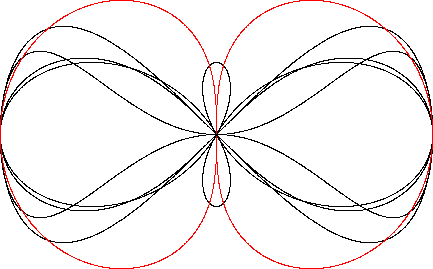Dipole
DIPOLE

Balmoral Software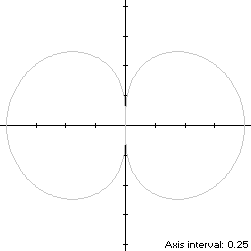The dipole is a figure-8 curve S with polar equationThis bisymmetric curve is traced out in a counterclockwise direction around the origin, starting from the right edge at (1,0). Its maximum height occurs at the points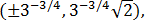which determine that the dipole is non-convex by the multiple local extrema test. The values of t at these points areand π -. The maximum width of the dipole is between the point pair (0,±1), so the width x height of its bounding rectangle is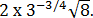### Metrics

We haveso by (L2), the perimeter of S is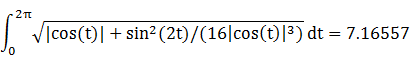and by (A2), the area of S is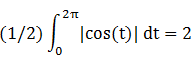### Convex Hull

The convex hull is created by connecting the extreme ordinate points with two horizontal line segments of length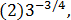as shown in blue in the left diagram below. By (L2), the perimeter of the convex hull is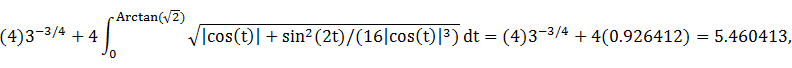which is about 24% shorter than that of the dipole.

The line segments of the convex hull create two isosceles triangles with the origin, each having an area of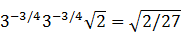By (A2), the area of the convex hull is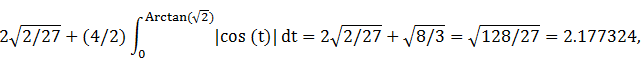which is about 9% more than the area of the dipole.

### Circumellipse

From Lemma B, we have
x(t)y(t) = r2(t)cos(t)sin(t) = |cos(t)|sin(2t)/2
This expression is maximized in the first quadrant at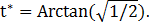The circumellipse dimensions are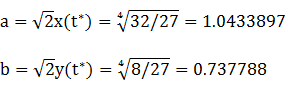For verification, we have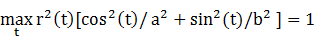### Incircle (lobe)

Consider the right lobe of the dipole, where -π/2 ≤ t < π/2. The lobe width 1 is smaller than its heightso a candidate for a circle inscribed in the lobe is one with a radius R and center abscissa c both equal to 1/2. For verification, we have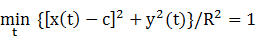### Inellipse (lobe)

Using z = 0 in Lemma E,
d/dt [x(t) - z]y(t) = d/dt |cos(t)|sin(2t)/2
We established in the preceding Circumellipse section that this expression has a zero in the first quadrant atThe corresponding coordinates are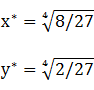We then have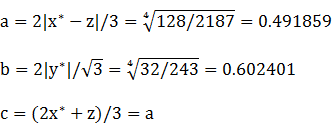For verification, we have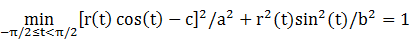The value of c is negated in the diagram below to display the inellipse separately in the left lobe.

### Summary Table

 Perimeter Area Centroid Figure Parameters Incircle (lobe) R = 1/2 3.141593 0.785398 (0.5,0) Inellipse (lobe)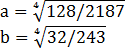3.446495 0.930842 (0.491859,0) Dipole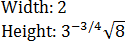7.16557 2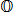Convex hull 5.460413 2.177324 Circumellipse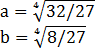5.636992 2.418399 Circumcircle R = 1 6.283185 3.141593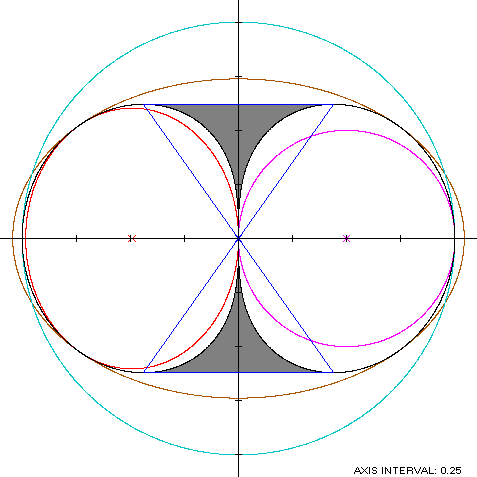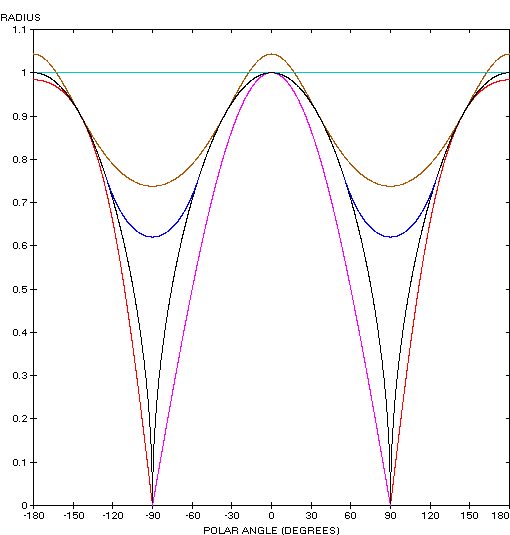Thong?

The dipole (red) is a member of a group of figure-8 curves described on these pages, including (inside to outside) the dumbbell curve, the bowtie, the Lemniscate of Bernoulli and the Lemniscate of Gerono: